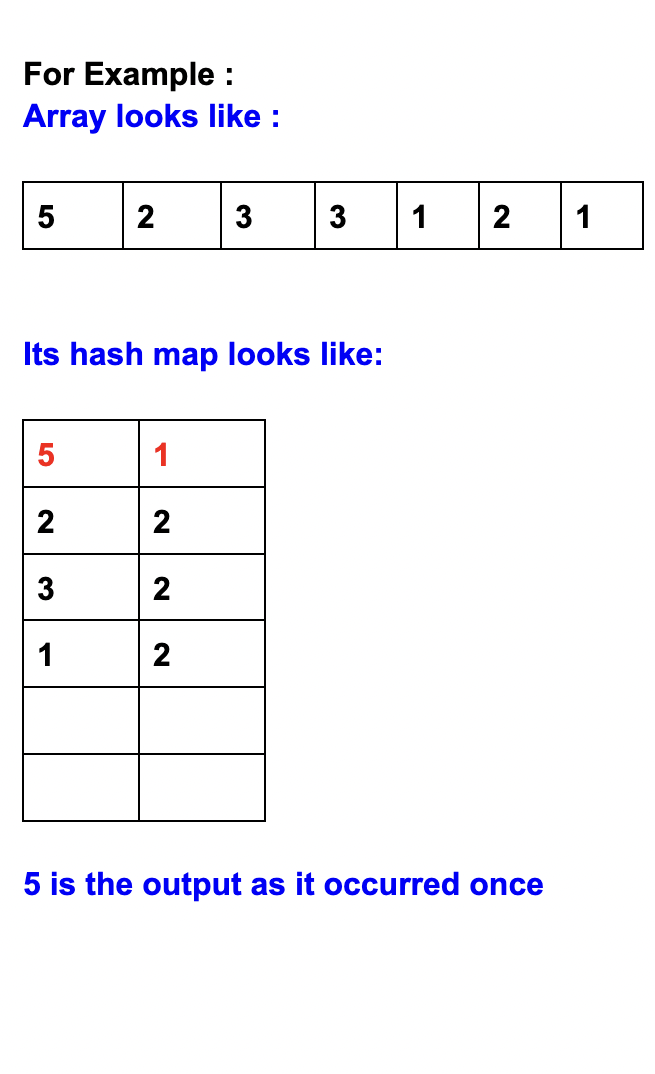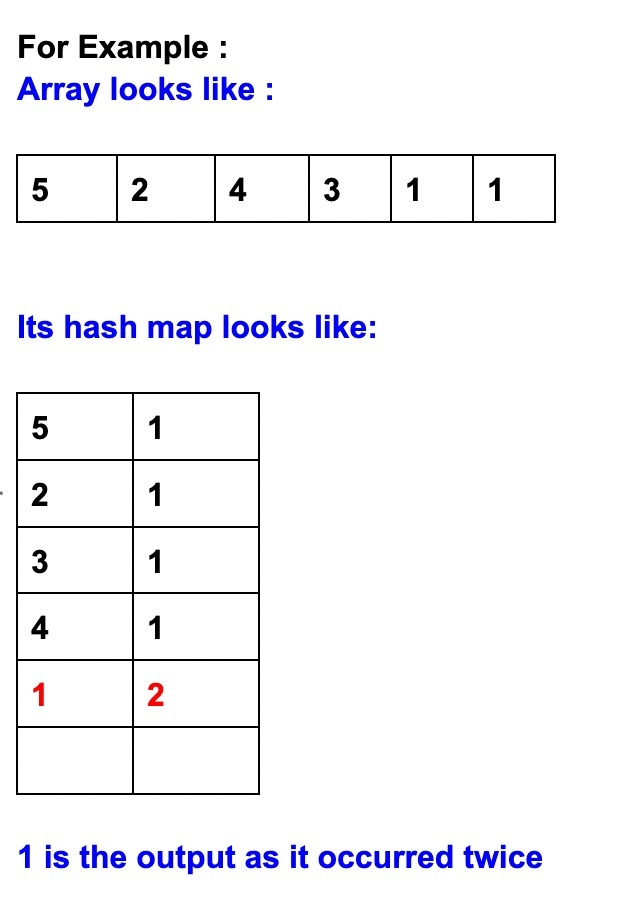# CANMRBL - Editorial

Author: Hasan Usmani
Tester: Abhishek Ghosh
Editorialist: Arghya Bera, Ekam Singh Ahuja

Simple

# PREREQUISITES

Observation, Frequency array

# PROBLEM

We are provided with a series of numbers that represent different colours, we need to check -
If the series having all duplicates pairs have any unique element
If the series having all unique have any duplicate element

# QUICK EXPLANATION

We can observe that only two types of data set are present.
First, all the elements are unique except one and that will be our outcome.
Second, all the elements are present in duplicate pairs except one and that will be our outcome.
Else, all other cases have -1 as their answer.

# EXPLANATION

1) We need a data structure that will hold the frequency of each number (colour).
2) This can be done by creating a hashmap which will have keys as A_{i} and frequency as the value.
3) Then we just need to identify which keys have different frequencies from other keys.
4) And we need to output that key.
5) Note that frequency can be only 1 and 2.

There are few cases to handle:

• If the number of keys having frequency 1 equal to 0 then no mistake is committed , hence the output is -1.
• If the number of keys having frequency 2 is equal to 0 then no mistake is committed , hence the output is -1.
• If the number of keys having frequency of one is greater than one and number of keys having frequency of two is greater than one then multiple mistakes are committed and output will be -1.
Example 1

**Example 2**

# Time complexity

O(N) or O(NlogN) depending upon implementation, for each test case.

# Solution

Setter's Solution
``````import java.util.*;
import java.io.*;

class Codechef  {
final private int BUFFER_SIZE = 1 << 16;
private DataInputStream din;
private byte[] buffer;

din = new DataInputStream(System.in);
buffer = new byte[BUFFER_SIZE];
}

public Reader(String file_name) throws IOException {
din = new DataInputStream(
new FileInputStream(file_name));
buffer = new byte[BUFFER_SIZE];
}

public String readLine() throws IOException {
byte[] buf = new byte; // line length
int cnt = 0, c;
while ((c = read()) != -1) {
if (c == '\n') {
if (cnt != 0) {
break;
} else {
continue;
}
}
buf[cnt++] = (byte)c;
}
return new String(buf, 0, cnt);
}

public int nextInt() throws IOException {
int ret = 0;
while (c <= ' ') {
}
boolean neg = (c == '-');
if (neg)
do {
ret = ret * 10 + c - '0';
} while ((c = read()) >= '0' && c <= '9');

if (neg)
return -ret;
return ret;
}

public long nextLong() throws IOException {
long ret = 0;
while (c <= ' ')
boolean neg = (c == '-');
if (neg)
do {
ret = ret * 10 + c - '0';
} while ((c = read()) >= '0' && c <= '9');
if (neg)
return -ret;
return ret;
}

public double nextDouble() throws IOException {
double ret = 0, div = 1;
while (c <= ' ')
boolean neg = (c == '-');
if (neg)

do {
ret = ret * 10 + c - '0';
} while ((c = read()) >= '0' && c <= '9');

if (c == '.') {
while ((c = read()) >= '0' && c <= '9') {
ret += (c - '0') / (div *= 10);
}
}

if (neg)
return -ret;
return ret;
}
private void fillBuffer() throws IOException {
BUFFER_SIZE);
buffer = -1;
}

private byte read() throws IOException {
fillBuffer();
return buffer[bufferPointer++];
}

public void close() throws IOException {
if (din == null)
return;
din.close();
}
}

public static void main(String[]args) throws Exception {

int T=sc.nextInt();

while(T--!=0)
{
int N=sc.nextInt();
ArrayList<Integer> list=new ArrayList<>();

for(int j=0;j<N;j++)
{
int ele=sc.nextInt();

}

find_duplicate_unique(list);

}

}

public static void find_duplicate_unique(ArrayList<Integer> arr)
{
HashMap<Integer,Integer> map=new HashMap<>();

int dup=0,uniq=0;
for(int i=0;i<arr.size();i++)
{
if(map.containsKey(arr.get(i)))
{dup++;
uniq--;
}
else
uniq++;

map.put(arr.get(i),map.getOrDefault(arr.get(i),0)+1);

}

if(uniq==arr.size()||uniq==0||uniq==dup)

else if(uniq>dup&&dup==1)
{
for(Map.Entry<Integer,Integer> set:map.entrySet())
{
if(set.getValue()>1)
{
break;
}
}
}
else if(uniq==1&&dup>uniq)
{
for(Map.Entry<Integer,Integer> set:map.entrySet())
{
if(set.getValue()==1)
{
break;
}
}

}

}

}
``````
Editorialist's Solution
``````#include <bits/stdc++.h>
using namespace std;

int main() {
int t;
cin>>t;
while(t--){
int n;
cin>>n;
int arr[n];
unordered_map<int, int> mp;
for(int i=0;i<n;i++){
cin>>arr[i];
mp[arr[i]]++;
}

int singles=0;
int pairs=0;
//ans storage
vector<int> pair;
vector<int> single;
unordered_map<int, int>::iterator it;
for(it = mp.begin() ; it!=mp.end() ; it++){
if((*it).second == 1){
single.push_back((*it).first);
singles++;
}
else{
pair.push_back((*it).first);
pairs++;
}
}
//cout<<pairs<<":"<<singles<<" ";

//handling -1 cases
if(singles==0||pairs==0){
cout<<-1<<endl;
continue;
}
if(singles>1&&pairs>1){
cout<<-1<<endl;
continue;
}
if(singles==pairs){
cout<<-1<<endl;
continue;
}
if(singles==1){
cout<<single<<endl;
}
if(pairs==1){
cout<<pair<<endl;
}

}
return 0;
}
``````